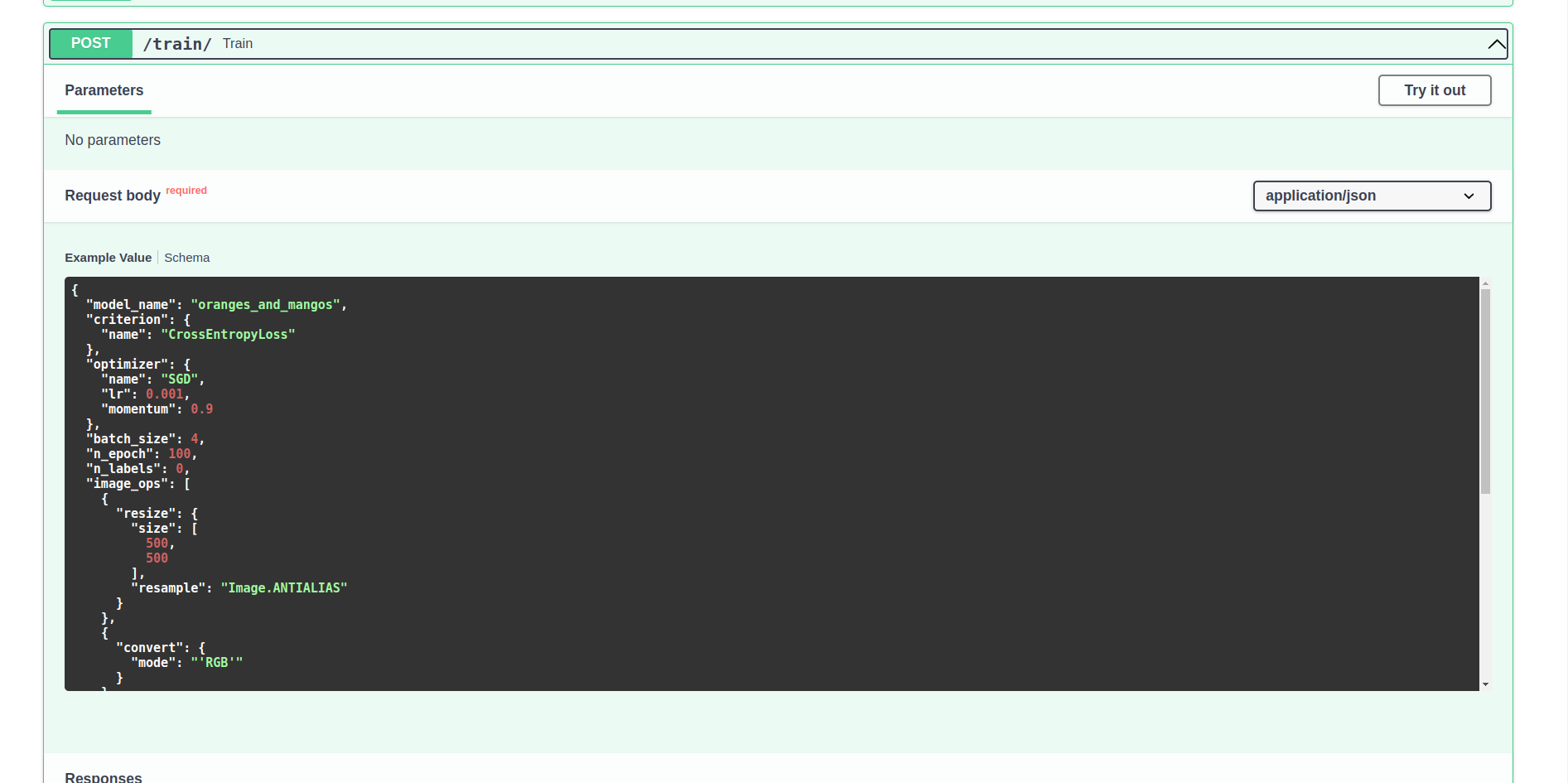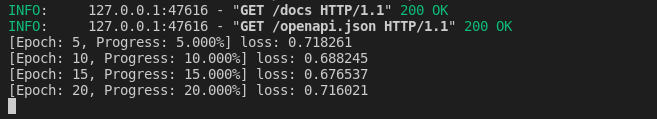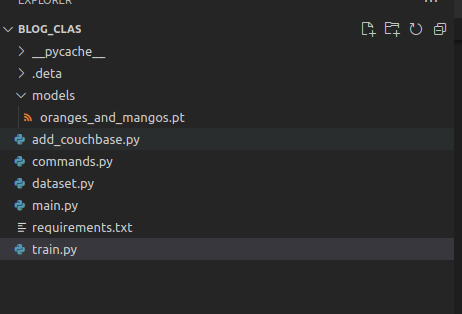This is a part of the series of blog posts related to Artificial Intelligence Implementation. If you are interested in the background of the story or how it goes:

On the previous weeks we explored how to create your own image dataset using SerpApi's Google Images Scraper API automatically. This week we will reshape the structure for training commands, data loading, and training to make everything completely efficient, and fully customizable.

## New Commands Structure for Automatic Training

On the past weeks, we have passed a command dictionary to our endpoint to automatically train a model. I have mentioned the changes I made when explaining the structre. Idea is to have more customizable fields over the commands dictionary in order to train and test your models at large. We will give custom control over everything except the model.

Also on previous weeks we have introduced a way to call a random image from Couchbase Server to use for training purposes. There are good and bad side effects to this approach. But, luckily, bad side effects can be controlled in upcoming updates.

Good part of fetching images from a Couchbase server randomly is its ability to operate without knowing dataset size, speed of operation, efficient storing, and future availibility for async fetching from a storage server. This last part could be crucial in reducing the time to train a model, which is a major problem in training big image classifiers. We will see if there will be any other bumps in the road to achieve general cuncurrent training.

Bad part of fetching images from a Couchbase server, for now, is that we won't have a separation between a training database or a testing database. We will randomly fetch images from the storage server, which also means we could train the network with two images twice. All of these side effects are vital only when using a small number of images. Considering SerpApi's Google Images Scraper API can provide up to 100 images per call, everything I just mentioned could be ignored in the scope of this endeavour. Maybe in the future, we can create another key within the image object in the Couchbase server to have a control based on the model you are training.

Let's take a look at the new command structure:

``````## /commands.py
from pydantic import BaseModel

class TrainCommands(BaseModel):
model_name: str = "oranges_and_mangos"
criterion: dict = {"name": "CrossEntropyLoss"}
optimizer: dict = {"name": "SGD", "lr": 0.001, "momentum": 0.9 }
batch_size: int = 4
n_epoch: int = 100
n_labels: int = None
image_ops: list = [{"resize":{"size": (500, 500), "resample": "Image.ANTIALIAS"}}, {"convert": {"mode": "'RGB'"}}]
transform: dict = {"ToTensor": True, "Normalize": {"mean": (0.5, 0.5, 0.5), "std": (0.5, 0.5, 0.5)}}
target_transform: dict = {"ToTensor": True}
label_names: list = ["Orange", "Mango"]
``````

As you can see, parameters of `criterion`, `optimizer` can be passed from the dictionary body now. Every key except `name` will be used as a parameter.
For `criterion` in this context, the object called will be:

``````CrossEntropyLoss()
``````

For optimizer:

``````SGD(lr = 0.001, momentum = 0.9)
``````

`image_ops` represent the image manipulation methods for `Image` class of the `PIL` library. Each element is in itself a function to be called:

``````Image.resize(size = (500,500), resample: Image.ANTIALIAS)
``````

and

``````Image.convert(mode = "RGB")
``````

Notice that to pass a string object we nest it in another apostrophe.

`transform` and `target_transform` will create an array with `torch.nn.transforms` to compose after transformations to be made to the image. `True` means an operation without a parameter. In our case:

``````transforms.ToTensor()
``````

For variables containing a dictionary, items in the dictionary will be called as parameters:

``````transforms.Normalize(mean = (0.5, 0.5, 0.5), std = (0.5, 0.5, 0.5))
``````

## Fetching Random Images from a Storage Server with Custom Dataset and Custom Dataloader

Here I had to introduce a way to read images from the database instead of local storage, and return them as tensors.

Also, another challenge was to ditch `torch.utils.data.DataLoader` and introduce a way to return images as tensors just like the class does. The reason was simple. `DataLoader` is using iteration whereas we need to call objects `batch_size` many times randomly and return them just like before.

Here is the refactored version of imports:

``````## /dataset.py
from torch.utils.data import Dataset
from torchvision import transforms
from commands import TrainCommands
from PIL import Image
import numpy as np
import warnings
import random
import base64
import torch
import io
``````

Let's initiate the Dataset object:

``````	def __init__(self, tc: TrainCommands, db: ImagesDataBase):
transform = tc.transform
target_transform = tc.target_transform
self.image_ops = tc.image_ops
self.label_names = tc.label_names
tc.n_labels = len(self.label_names)
self.db = db

def create_transforms(transforms_dict):
transforms_list = []
for operation in transforms_dict:
if type(transforms_dict[operation]) == bool:
string_operation = "transforms.{}()".format(operation)
elif type(transforms_dict[operation]) == dict:
string_operation = "transforms.{}(".format(operation)
for param in transforms_dict[operation]:
string_operation = string_operation + "{}={},".format(param, transforms_dict[operation][param])
string_operation = string_operation[0:-1] + ")"
transforms_list.append(eval(string_operation))
return transforms_list

if transform != None:
transforms_list = create_transforms(transform)
self.transform = transforms.Compose(transforms_list)
else:
self.transform == False

if target_transform != None:
transforms_list = create_transforms(target_transform)
self.target_transform = transforms.Compose(transforms_list)
else:
self.target_transform == False
``````

Notice that we don't have a type of operation (training, testing) to pass now. Instead, we pass a database object. Also all the initialization of elements are now changed to work with new command structure.

`create_transforms` function is responsible for recursive object creation in `transforms`. We then add each creation into an array, and compose them in the main function.

Instead of iteration function (`__get_item__`), we now refer to another function:

``````	def get_random_item(self):
while True:
try:
random_label_idx = random.randint(0,len(self.label_names) - 1)
label = self.label_names[random_label_idx]

label_arr = np.full((len(self.label_names), 1), 0, dtype=float)
label_arr[random_label_idx] = 1.0

image_dict = self.db.random_lookup_by_classification(self.db(),label)["\$1"]
buf = base64.b64decode(image_dict['base64'])
buf = io.BytesIO(buf)
img = Image.open(buf)
break
except:
print("Couldn't fetch the image, Retrying with another random image")
``````

Putting this in while True could seem brutal. But considering we have images in the database, we won't have any problems with calling a new image randomly. I encountered a few minor problems with some of the images in Couchbase storage. I didn't investigate further. It could be shaped better in the future.

But for now, we call a random `label` from `label_names` we provided in the command dictionary. Then we make a one-hot dictionary of the label.
Finally, we call for the image from the database. `["\$1"]` part is admittably, another lazy programming error I made in previous weeks' blog post. Not a vital one though. We take the `base64` of the image, decode it into `bytes`, store it in `BytesIO` object, and then read it with `Image`.

On the next part of the function, we make operations on the image based on the entries in commands dictionary:

``````		if self.image_ops != None:
for op in self.image_ops:
for param in op:
if type(op[param]) == bool:
string_operation = "img.{}()".format(param)
elif type(op[param]) == dict:
string_operation = "img.{}(".format(param)
for inner_param in op[param]:
string_operation = string_operation + "{}={},".format(inner_param, op[param][inner_param])
string_operation = string_operation[0:-1] + ")"

with warnings.catch_warnings():
warnings.simplefilter("ignore")
img = eval(string_operation)
``````

Then comes the transformations for torch we defined in initializing the Dataset:

``````		if not self.transform	== False:
img = self.transform(img)

if not self.target_transform == False:
label = self.target_transform(label_arr)

return img, label
``````

Finally, we return the image and the label.

For dataloader, we pass commands dictionary, dataset object, and Couchbase Database adaptor:

``````class CustomImageDataLoader:
def __init__(self, tc: TrainCommands, cid: CustomImageDataset, db: ImagesDataBase):
self.batch_size = tc.batch_size
self.cid = cid(tc, db)
``````

For iterating a training, we resort to a longer method now:

``````	def iterate_training(self):
train_features = []
train_labels = []
for i in range(0,self.batch_size):
img, label = self.cid.get_random_item()
train_features.append(img)
train_labels.append(label)
train_features = [t.numpy() for t in train_features]
train_features = np.asarray(train_features, dtype='float64')
train_features = torch.from_numpy(train_features).float()

train_labels = [t.numpy() for t in train_labels]
train_labels = np.asarray(train_labels, dtype='float64')
train_labels = torch.from_numpy(train_labels).float()

return train_features, train_labels
``````

We create a list of images and labels. And then convert them `numpy.ndarray`, then convert them back to float64 pytorch tensors. Finally, we return them at each epoch.

## Training Process

I changed the way we train the model from iterating through a dataset to only epochs with random fetchings of images. Also, I added support for training using gpu if it is supported. Here are the updated imports:

``````## /train.py
from commands import TrainCommands
import torch.nn.functional as F
import torch.nn as nn
import torch
``````

I didn't change anything in the model, although, I didn't have the time to explain the manual calculation I made there into an automatic one with an explanation. That will be for the next weeks:

``````class CNN(nn.Module):
def __init__(self, tc: TrainCommands):
super().__init__()
n_labels = tc.n_labels
self.conv1 = nn.Conv2d(3, 6, 5)
self.pool = nn.MaxPool2d(2, 2)
self.conv2 = nn.Conv2d(6, 16, 5)
self.flatten = nn.Flatten(start_dim=1)
self.fc1 = nn.Linear(16*122*122, 120) # Manually calculated I will explain next week
self.fc2 = nn.Linear(120, 84)
self.fc3 = nn.Linear(84, n_labels) #unique label size

def forward(self, x):
x = self.pool(F.relu(self.conv1(x)))
x = self.pool(F.relu(self.conv2(x)))
x = self.flatten(x)
x = F.relu(self.fc1(x))
x = F.relu(self.fc2(x))
x = self.fc3(x)
return x
``````

Let's initialize our training class in accordance with the information in the commands dictionary:

``````class Train:
def __init__(self, tc: TrainCommands, cnn: CNN, cidl: CustomImageDataLoader, cid: CustomImageDataset, db: ImagesDataBase):
self.cnn = cnn(tc)

criterion_dict = tc.criterion
criterion_name = criterion_dict.pop('name')
if len(criterion_dict.keys()) == 0:
self.criterion = getattr(nn, criterion_name)()
else:
self.criterion = getattr(nn, criterion_name)(**criterion_dict)

optimizer_dict = tc.optimizer
optimizer_name = optimizer_dict.pop('name')
if len(optimizer_dict.keys()) == 0:
self.optimizer = getattr(torch.optim, optimizer_name)(self.cnn.parameters())
else:
self.optimizer = getattr(torch.optim, optimizer_name)(self.cnn.parameters(), **optimizer_dict)
self.n_epoch = tc.n_epoch
self.model_name = tc.model_name
``````

As you can see, `optimizer`, and `criterion` are created from the variables we provide. Also, we need to increase `n_epochs` which is the number of epochs we iterate to train the model since we don't go through full training dataset now. So, in the end, if we provide the epochs to be `100`, it'll fetch 100 random images from the storage at each epoch, and train on it.

Here's the main training process:

``````	def train(self):
device = torch.device('cuda:0' if torch.cuda.is_available() else 'cpu')

for epoch in range(self.n_epoch):  # loop over the dataset multiple times
running_loss = 0.0
inputs, labels = inputs.to(device), labels.to(device)
if torch.cuda.is_available():
self.cnn.cuda()
outputs = self.cnn(inputs).to(device)
else:
outputs = self.cnn(inputs)
loss = self.criterion(outputs, labels.squeeze())

loss.backward()
self.optimizer.step()
running_loss = running_loss + loss.item()
if epoch % 5 == 4:
print(f'[Epoch: {epoch + 1}, Progress: {((epoch+1)*100/self.n_epoch):.3f}%] loss: {running_loss:.6f}')
running_loss = 0.0
torch.save(self.cnn.state_dict(), "models/{}.pt".format(self.model_name))
``````

As you can see, we fetch a random image at every epoch, and train on it using cpu or gpu, whichever is available. We then report on the process, and in the end, save it using designated model name:

## Showcase

If we run the server, and head to `localhost:8000/docs`, to try out the new `/train` endpoint with default commands:As soon as we execute, we can observe the training process in the terminal:Once the training ends, the model will be saved with designated name:## Conclusion

I am grateful to the brilliant people of SerpApi for making this blog post post possible, and I am grateful to the reader for their attention. We haven't gone through some keypoints I mentioned in prior weeks. However, we have cleared the path for some crucial parts. In the following weeks, we will discuss how to make the model customizable, and callable from a file name, cuncurrent calls to SerpApi's Google Images Scraper API, and create a comparative test structure between different models.